# OECD Nuclear power plants data analysis 2 - Getting Confidence Interval using R with infer packageUnsplashEean Chenが撮影した写真

This post is following of the above post.
I will calculate confidence interval in this post.

There are two ways to calclulate confidence interval, one is bootstrap method and the other is traditional(formula) method.

To do bootstrap, I load infer package.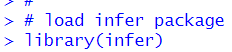Firstly, let's to bootstrap.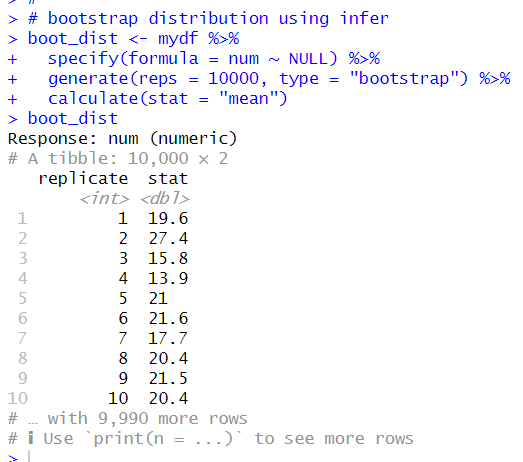I made bootstrap distribution of mean.

let's get averge num.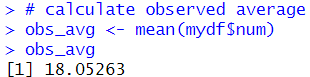Let's visualize bootstrap ditribution and observed average.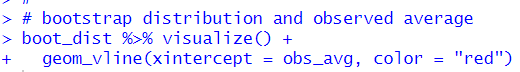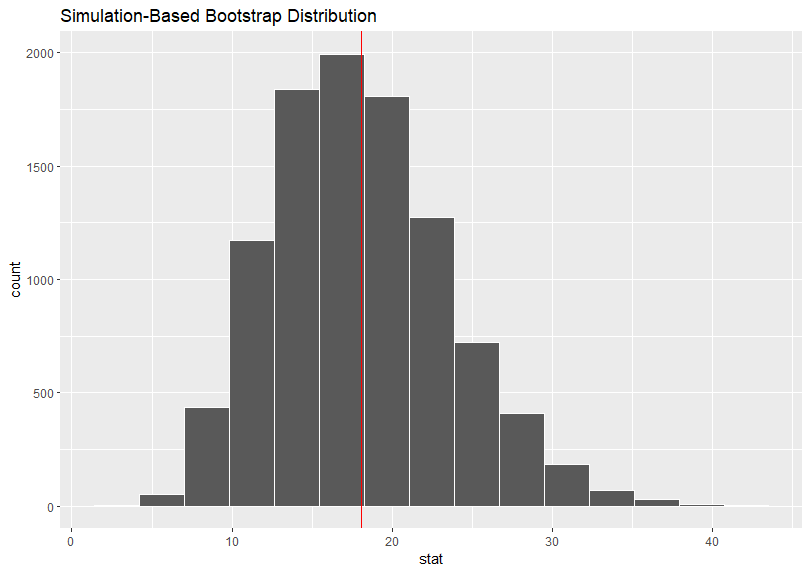To get confidence interval, I use get_confidence_interval() frunction.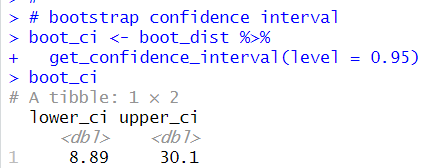So, with bootstrap method, 95% confidence interval is 8.89 ~ 30.1.

Then, let's calculate confidence interval using traditional(formula) method.

We need some components to calculate confidence interval.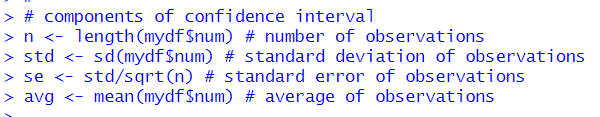then, I can calculate confidence interval.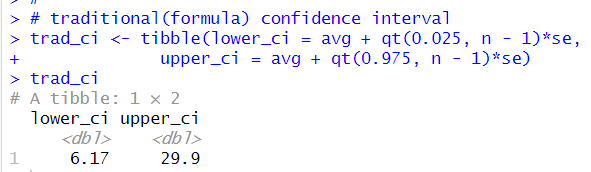Using t.test() function is the easiest way to get traditional confidence interval.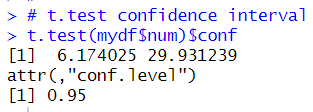Now, let's compare bootstrap confidence interval and traditional(formula) confidence interval.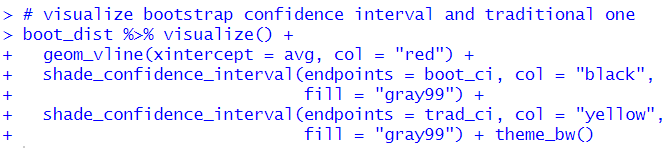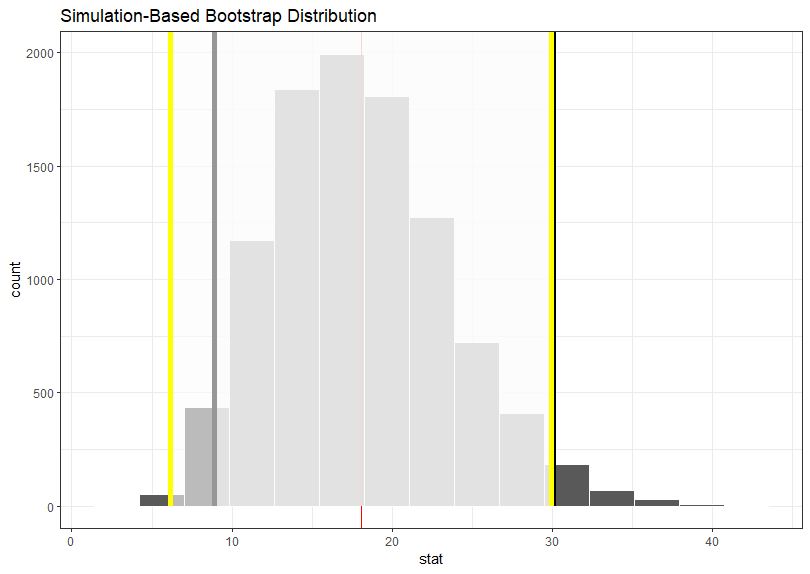I see bootstrap confidence interval is norrower than traditional(formula base) confidence interval.

That's it. Thank you!

Next post is